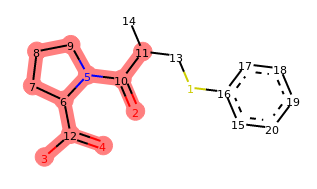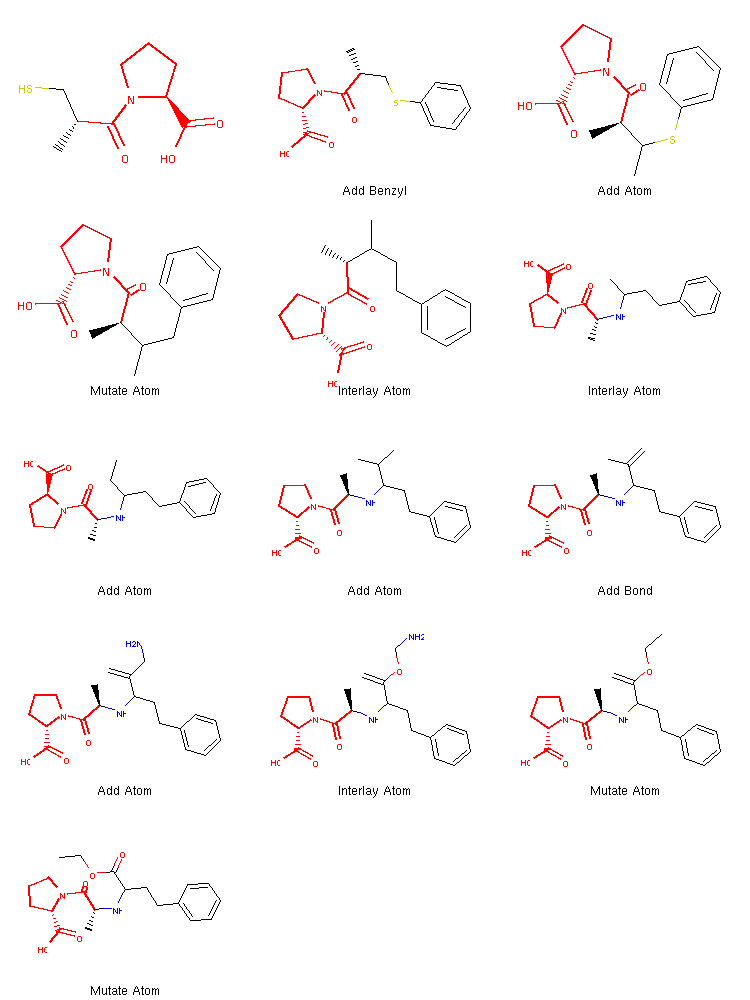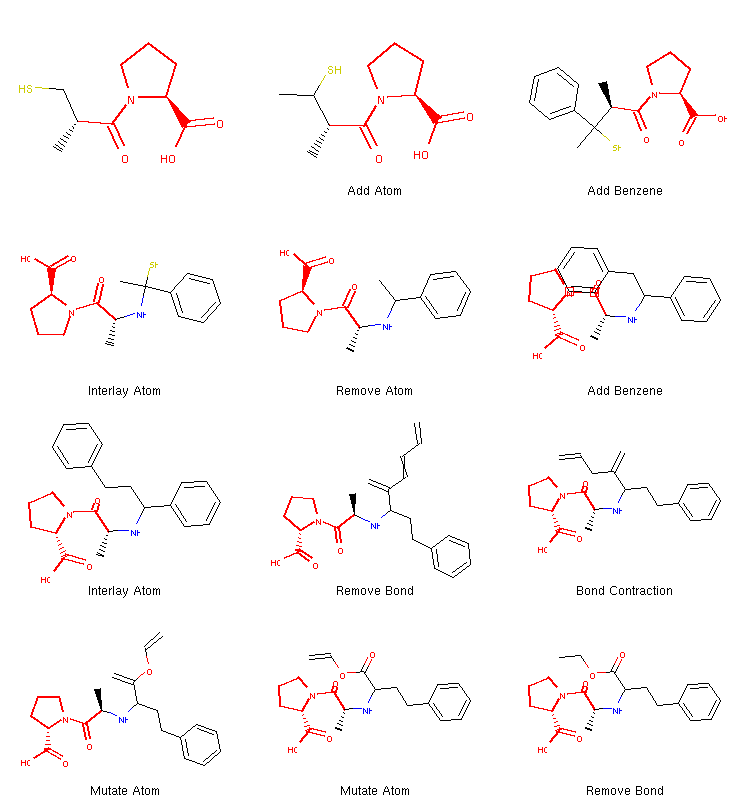## 2.4.1. Customized Morphing Operators¶

Let us now get back to the concept of chemical operators and show an example implementation of a simple new operator, as we promised while we talked about the basics of molecular morphing in the very first section of this tutorial.

First, about the operator itself. We will define a new chemical operator that we would like to incorporate into the workflow we developed previously. This operator will give us the ability to take ‘shortcuts’ in chemical space by adding certain fragments rather than just atoms. With a little help from the RDKit library, such operator could be defined as follows:

  1 2 3 4 5 6 7 8 9 10 11 12 13 14 15 16 17 18 19 20 21 22 23 24 25 26 27 28 29 30 31 32 33 34 35 36 37 38 39 40 41 42 43 44 45 from rdkit import Chem from molpher.core import MolpherMol, MolpherAtom from molpher.core.morphing.operators import MorphingOperator from molpher.random import get_random_number class AddFragment(MorphingOperator): def __init__(self, fragment, open_atoms_frag, oper_name): super(AddFragment, self).__init__() self._name = oper_name self._fragment = fragment self._open_atoms_frag = open_atoms_frag self._orig_rdkit = None self._open_atoms = [] def setOriginal(self, mol): super(AddFragment, self).setOriginal(mol) if self.original: self._orig_rdkit = self.original.asRDMol() self._open_atoms = [] for atm_rdkit, atm_molpher in zip(self._orig_rdkit.GetAtoms(), self.original.atoms): free_bonds = atm_rdkit.GetImplicitValence() if free_bonds >= 1 and not (MolpherAtom.NO_ADDITION & atm_molpher.locking_mask): self._open_atoms.append(atm_rdkit.GetIdx()) def morph(self): combo_mol = Chem.EditableMol(Chem.CombineMols( self._orig_rdkit , self._fragment )) atom_orig = self._open_atoms[get_random_number(0, len(self._open_atoms)-1)] atom_frag = len(self.original.atoms) + self._open_atoms_frag[get_random_number(0, len(self._open_atoms_frag)-1)] combo_mol.AddBond(atom_orig, atom_frag, order=Chem.rdchem.BondType.SINGLE) combo_mol = combo_mol.GetMol() Chem.SanitizeMol(combo_mol) ret = MolpherMol(other=combo_mol) for atm_ret, atm_orig in zip(ret.atoms, self.original.atoms): atm_ret.locking_mask = atm_orig.locking_mask return ret def getName(self): return self._name 

A new chemical operator must implement the MorphingOperator abstract class and define three methods:

1. setOriginal() – used to set the structure that will be modified during morphing. Its purpose is to figure out what atoms in the molecule can be changed and what restrictions apply. In our example, we just find all atoms that have at least one implicit bond to a hydrogen atom. We save the indices of such atoms in the _open_atoms member of our AddFragment class. Note that we also made sure that no locked atoms were added to the list as well.

2. morph() – This is the method where the original molecule is changed to a new one. In our example, we randomly pick one atom from the original molecule and one from our fragment. Then we connect them. After we are done, we make sure to sanitize the result and also transfer any locks from the original molecule to the new one.

3. getName() – This method is also required to implement and should return a string with the name of the operator. This is then saved to the parent_operator member of the MolpherMol instance returned by the morph method.

Let’s test if our new operator works as expected:

 1 2 3 4 5 captopril = MolpherMol("captopril.sdf") frag = Chem.MolFromSmiles('c1ccccc1') add_frag = AddFragment(frag, , "Add Benzene") add_frag.setOriginal(captopril) depict(add_frag.morph()) 

Output:Indeed, our operator seems to be doing what we designed it to do. If you run the last line of this code a few times, you should see different variations of captopril with a benzene ring attached at random positions. The locked positions should always be excluded.

We omit the source of the depict method, which is too long and not relevant at the moment. It is defined in the example Jupyter Notebook, though ( available for download or rendered).

The only thing that remains is to use our operator in the context of an exploration tree. Every tree has the morphing_operators property which stores the morphing operators currently in use. All we need to do, is to write a new list of operators and include the one we just defined:

 1 2 3 4 5 6 from molpher.core import ExplorationTree as ETree captopril = MolpherMol("captopril.sdf") enalapril = MolpherMol("O=C(O)[CH]2N(C(=O)[CH](N[CH](C(=O)OCC)CCc1ccccc1)C)CCC2") tree = ETree.create(source=captopril, target=enalapril) tree.morphing_operators = tree.morphing_operators + (add_frag,) 

Then we can run the exploration as before (Listing 2.8):

  1 2 3 4 5 6 7 8 9 10 11 12 13 14 15 16 17 18 19 20 21 22 23 24 25 26 27 class FindClosest: def __init__(self): self.closest_mol = None self.closest_distance = None def __call__(self, morph): if not self.closest_mol or self.closest_distance > morph.dist_to_target: self.closest_mol = morph self.closest_distance = morph.dist_to_target closest_info = FindClosest() while not tree.path_found: tree.generateMorphs() tree.sortMorphs() tree.filterMorphs() tree.extend() tree.prune() tree.traverse(closest_info) print('Generation #', tree.generation_count, sep='') print('Molecules in tree:', tree.mol_count) print( 'Closest molecule to target: {0} (Tanimoto distance: {1})'.format( closest_info.closest_mol.getSMILES() , closest_info.closest_distance ) ) 

Output (some contents omitted):

Generation #1
Molecules in tree: 21
Closest molecule to target: CC(CSC1=CC=CC=C1)C(=O)N1CCCC1C(=O)O (Tanimoto distance: 0.484375)
Generation #2
Molecules in tree: 105
Closest molecule to target: CC(CCC1=CC=CC=C1)C(=O)N1CCCC1C(=O)O (Tanimoto distance: 0.3035714285714286)
Generation #3
Molecules in tree: 205
Closest molecule to target: CC(CCC1=CC=CC=C1)C(=O)N1CCCC1C(=O)O (Tanimoto distance: 0.3035714285714286)
...
Generation #10
Molecules in tree: 787
Closest molecule to target: C=C(CC)C(CCC1=CC=CC=C1)NC(C)C(=O)N1CCCC1C(=O)O (Tanimoto distance: 0.21666666666666667)
Generation #11
Molecules in tree: 811
Closest molecule to target: CC(NC(CCC1=CC=CC=C1)C(=O)OCN)C(=O)N1CCCC1C(=O)O (Tanimoto distance: 0.13793103448275867)
Generation #12
Molecules in tree: 838
Closest molecule to target: CCOC(=O)C(CCC1=CC=CC=C1)NC(C)C(=O)N1CCCC1C(=O)O (Tanimoto distance: 0.0)


There is literally no change to our morphing code. We only added one more operator to the tree prior to running it. We can see that the algorithm only needs 12 iterations to converge this time (the original algorithm above needed 44). Therefore, our shortcut was successful and we managed to spare the algorithm the tedious aromatic ring creation we commented on before.

Let’s take a look at the generated path now:

show_mol_grid(tree.fetchPathTo(tree.params['target']))


Output:We can see that once the benzene ring is incorporated at the very beginning, the algorithm quickly finds the operations needed to create the target structure.

Since there is some randomization involved in this process, running the algorithm for a second time results in a different path:We can see that this time the algorithm decided to take a different route. It added two benzene rings quite early and then proceeded to break one of them down to create the final structure. Therefore, running the same algorithm multiple times can lead to different coverage of chemical space and different structures can be discovered this way.

## 2.4.2. Tree Operations¶

In the previous section, we used some methods of the ExplorationTree to generate new morphs and extend it, but also to prune it. These methods, however, have one thing in common. They work with a single exploration tree instance and change it somehow. Therefore, their functionality can be defined with an interface and that is what we will cover in this section, the tree operation interface and how to use it.

We call every action that is performed on an exploration tree a tree operation. This concept is represented in the library with the TreeOperation abstract class and it becomes useful when we need to control several exploration trees at once or if we just prefer to separate the tree itself from the logic of our exploration algorithm.

Note

Tree operations were mainly created to house different parts of the exploration algorithm and to make them more encapsulated and configuration more intuitive. However, this transition is still far from complete so most of the built-in operations take their parameters from the params property, which is defined globally for the tree in question. Most of these parameters will eventually be encapsulated by their respective operations, though.

When we have defined our own operation, we can run it on a tree by supplying it to the runOperation() method. Here is an example of how to define a customized filtering procedure (similar to the one used before) and incorporate it into an exploration algorithm:

Listing 2.12 Using tree operations to define an iteration of a simple chemical space exploration algorithm.
  1 2 3 4 5 6 7 8 9 10 11 12 13 14 15 16 17 18 19 20 21 22 23 24 25 26 27 28 29 30 31 32 33 34 35 36 37 38 39 40 41 42 43 44 import molpher from molpher.core.operations import * from molpher.core import MolpherMol, ExplorationTree as ETree class MyFilterMorphs(TreeOperation): """ A custom tree operation that accepts only the first ten morphs after the list of candidates is sorted. """ def __call__(self): """ This method is called automatically by the tree. The tree this operation is being run on is accessible from the 'tree' member of the class. """ self.tree.candidates_mask = [ True if idx < 20 and self.tree.candidates[idx].sascore < 6 else False for idx, x in enumerate(self.tree.candidates_mask) ] cocaine = MolpherMol('CN1[CH]2CC[CH]1[CH](C(OC)=O)[CH](OC(C3=CC=CC=C3)=O)C2') procaine = MolpherMol('O=C(OCCN(CC)CC)c1ccc(N)cc1') tree = ETree.create(source=cocaine, target=procaine) # create the tree # list of tree operations, defines one iteration iteration = [ GenerateMorphsOper() , SortMorphsOper() , MyFilterMorphs() # our custom filtering procedure , ExtendTreeOper() , PruneTreeOper() ] # apply the operations in the list one by one for oper in iteration: tree.runOperation(oper) # observe the results print(tree.generation_count) print(len(tree.leaves)) 

Output:

1
18


This and other advanced examples are included in a Jupyter Notebook which can be downloaded from here or viewed directly.

Except for the source and target molecule, this algorithm is similar to what we have seen before, but this time we used operations instead of calling the corresponding methods on the tree. We used a customized operation for the filtering step by creating a subclass of the TreeOperation abstract class and we overrode its __call__() method with the implementation we want.

Each operation can have a tree associated with it, but it is not necessary (we had no problems initializing the operations without a tree in the previous example). We can verify if a tree is associated with an operation by calling its getTree() method or accessing the TreeOperation.tree attribute of the class. If there is no tree associated with the instance, they both return None. We can set the tree to operate on by writing into the TreeOperation.tree attribute or calling the TreeOperation.setTree method. Then the operation becomes callable (calling it will result in a RuntimeError).

### 2.4.2.1. Built-in Operations¶

Warning

This section is not yet complete because most of these operations will change their interface in the feature. We only describe the TraverseOper class, which has more practical use than the others and its interface will not undergo much change in the future. For the other operations, we kindly refer the reader to their respective documentation pages.

In this section, we describe the few operations the library inherited from Molpher:

They are all derived from TreeOperation and contain the full set of operations performed on a tree in the original Molpher algorithm as published in . Therefore, the original algorithm can be implemented using those operations.

#### 2.4.2.1.1. Traversing the Tree¶

A special place among these operations belongs to the TraverseOper class. It does not directly implement a part of a morphing algorithm, but serves as a means of traversing molecules in a tree and reading/modifying them as needed:

Listing 2.13 Traversing the tree using a callback.
  1 2 3 4 5 6 7 8 9 10 11 12 13 14 15 16 17 18 19 20 21 22 23 24 25 26 27 from molpher.core.operations.callbacks import TraverseCallback class MyCallback(TraverseCallback): """ This callback just prints some information about the molecules in the tree. """ def __call__(self, morph): """ Method called on each morph in the tree -- starting from the root to leaves. """ if not morph.getParentSMILES(): print("# Root #") else: print('# Morph #') print('Parent:', morph.getParentSMILES()) print('SMILES: ', morph.getSMILES()) print('Descendents: ', morph.getDescendants()) callback = MyCallback() # initialize a callback traverse = TraverseOper(callback=callback) # attach it to a tree traversal operation tree.runOperation(traverse) # run the operation 

Output:

# Root #
SMILES:  COC(=O)C1C2CCC(CC1OC(=O)C1=CC=CC=C1)N2C
Descendents:  ('COC(=O)C1C(COC(=O)C2=CC=CC=C2)C2CCC1N2C', 'COC(=O)C1C2CCC(CC1OC(=O)C1=CC=C(N)C=C1)N2C', 'COC(=O)CC1CCC(CCOC(=O)C2=CC=CC=C2)N1C')
# Morph #
Parent: COC(=O)C1C2CCC(CC1OC(=O)C1=CC=CC=C1)N2C
# Morph #
Parent: COC(=O)C1C2CCC(CC1OC(=O)C1=CC=CC=C1)N2C
SMILES:  COC(=O)C1C(COC(=O)C2=CC=CC=C2)C2CCC1N2C
Descendents:  ()
# Morph #
Parent: COC(=O)C1C2CCC(CC1OC(=O)C1=CC=CC=C1)N2C
SMILES:  COC(=O)C1C2CCC(CC1OC(=O)C1=CC=C(N)C=C1)N2C
SMILES:  COC(=O)CC1CCC(CCOC(=O)C2=CC=CC=C2)N1C
Descendents:  ()
Descendents:  ()


Note

The tree traversal algorithm uses multiple threads. Therefore, the output might appear a little messy.

In Listing 2.13 we derive from the TraverseCallback class, an abstract class with an abstract method __call__(). This method takes one argument, which is a MolpherMol instance of a molecule in the tree. We need to override this method in our derived class in order to implement our own behaviour.

The callback is then associated with a TraverseOper instance, which can be run on a tree as any other tree operation. When the operation is run it traverses the tree from the root to the leaves and injects every molecule it encounters into our implementation of the __call__() method.

Note

We can also pass a SMILES string to the TraverseOper constructor. In that case, a subtree will be traversed using the specified molecule as the root of the subtree.

There is also a much more convenient way to traverse the tree. Because, the ExplorationTree class implements the molpher.core.ExplorationTree.ExplorationTree.traverse() method, we can simply take any python callable and use it instead of the __call__() method. However, under the hood it does the same thing as we did in Listing 2.13. Therefore, the above code can be turned into:

Listing 2.14 Traversing the tree using a callback – the simple version.
  1 2 3 4 5 6 7 8 9 10 11 12 13 14 15 16 def process(morph): """ Prints some information about the molecules in the tree. """ if not morph.getParentSMILES(): print("# Root #") else: print('# Morph #') print('Parent:', morph.getParentSMILES()) print('SMILES: ', morph.getSMILES()) print('Descendents: ', morph.getDescendants()) tree.traverse(process) # use the traverse method to run the callback function 

Output:

# Root #
SMILES:  COC(=O)C1C2CCC(CC1OC(=O)C1=CC=CC=C1)N2C
Descendents:  ('COC(=O)C1C(COC(=O)C2=CC=CC=C2)C2CCC1N2C', 'COC(=O)C1C2CCC(CC1OC(=O)C1=CC=C(N)C=C1)N2C', 'COC(=O)CC1CCC(CCOC(=O)C2=CC=CC=C2)N1C')
# Morph #
Parent: COC(=O)C1C2CCC(CC1OC(=O)C1=CC=CC=C1)N2C
SMILES:  COC(=O)CC1CCC(CCOC(=O)C2=CC=CC=C2)N1C
Descendents:  ()
# Morph #
Parent: COC(=O)C1C2CCC(CC1OC(=O)C1=CC=CC=C1)N2C
SMILES:  COC(=O)C1C2CCC(CC1OC(=O)C1=CC=C(N)C=C1)N2C
Descendents:  ()
# Morph #
Parent: COC(=O)C1C2CCC(CC1OC(=O)C1=CC=CC=C1)N2C
SMILES:  COC(=O)C1C(COC(=O)C2=CC=CC=C2)C2CCC1N2C
Descendents:  ()


## 2.4.3. Tree Templates and Snapshots¶

We don’t always have to initialize morphing parameters by hand. We can use an XML template instead. Here is an example of a template file (you can download this one from here):

Listing 2.15 A complete XML template file.
  1 2 3 4 5 6 7 8 9 10 11 12 13 14 15 16 17 18 19 20 21 22 23 24 25 26 27 28 29 30  CN1[C@H]2CC[C@@H]1[C@@H](C(=O)OC)[C@@H](OC(=O)c1ccccc1)C2 O=C(OCCN(CC)CC)c1ccc(N)cc1 FP_MORGAN SC_TANIMOTO 2 50 100 80 150 0.15 1500 2 0.0 500.0 

An XML template is similar to a configuration file and can be loaded just like a snapshot (see Listing 2.17), but the resulting tree will only contain the source molecule as its root.

Listing 2.16 Loading a template and saving a built tree as a XML snapshot.
  1 2 3 4 5 6 7 8 9 10 11 12 13 14 15 16 17 18 19 20 template_file = 'cocaine-procaine-template.xml' tree = ETree.create(template_file) print(tree.params) # apply the tree operations for oper in iteration: tree.runOperation(oper) print( sorted( # grab the new leaves as a list sorted according to their distance from target [ (x.getSMILES(), x.getDistToTarget()) for x in tree.leaves ], key=lambda x : x ) ) # save the tree in a snapshot file tree.save('snapshot.xml') 

Output:

The new iteration has been created from template:
source: CN1[C@H]2CC[C@@H]1[C@@H](C(=O)OC)[C@@H](OC(=O)c1ccccc1)C2
target: O=C(OCCN(CC)CC)c1ccc(N)cc1

{
'max_morphs_total': 1500,
'far_close_threshold': 0.15,
'weight_max': 500.0,
'close_produce': 150,
'fingerprint': 'MORGAN',
'accept_min': 50,
'source': 'COC(=O)C1C2CCC(CC1OC(=O)C1=CC=CC=C1)N2C',
'target': 'CCN(CC)CCOC(=O)C1=CC=C(N)C=C1',
'weight_min': 0.0,
'non_producing_survive': 2,
'accept_max': 100,
'operators': (
'BOND_CONTRACTION',
'BOND_REROUTE',
'INTERLAY_ATOM',
'MUTATE_ATOM',
'REMOVE_ATOM',
'REMOVE_BOND'
),
'far_produce': 80,
'similarity': 'TANIMOTO'
}
Generated 66 morphs.
[('CN1C2CCC1C(C(=O)OCN)C(OC(=O)C1=CC=CC=C1)C2', 0.7777777777777778), ('CCN1C2CCC1C(C(=O)OC)C(OC(=O)C1=CC=CC=C1)C2', 0.7936507936507937), ('CN1C2CCC1C(C(=O)ON)C(OC(=O)C1=CC=CC=C1)C2', 0.8064516129032258)]


In the above example we loaded an XML template, created a tree from it, extended the tree and serialized it as a snapshot. We can see that all the parameters are the same as in the XML template and that the resulting tree can be built using the same list of operations as in Listing 2.12.

In Listing 2.16 we also serialized our tree instance to disk with the save() method. The saved tree can be later reconstructed with the create() factory method:

  1 2 3 4 5 6 7 8 9 10 new_tree = ETree.create('snapshot.xml') # create a new tree from the saved snapshot print(new_tree.params) print( sorted( # grab the leaves in the created tree (these should be the same as those in the original tree) [ (x.getSMILES(), x.getDistToTarget()) for x in new_tree.leaves ], key=lambda x : x ) ) 

Output:

Snapshot successfully created from: snapshot.xml
{
'max_morphs_total': 1500,
'far_close_threshold': 0.15,
'weight_max': 500.0,
'close_produce': 150,
'fingerprint': 'MORGAN',
'accept_min': 50,
'source': 'COC(=O)C1C2CCC(CC1OC(=O)C1=CC=CC=C1)N2C',
'target': 'CCN(CC)CCOC(=O)C1=CC=C(N)C=C1',
'weight_min': 0.0,
'non_producing_survive': 2,
'accept_max': 100,
'operators': (
'BOND_CONTRACTION',
'BOND_REROUTE',
'INTERLAY_ATOM',
'MUTATE_ATOM',
'REMOVE_ATOM',
'REMOVE_BOND'
),
'far_produce': 80,
'similarity': 'TANIMOTO'
}
[('CN1C2CCC1C(C(=O)OCN)C(OC(=O)C1=CC=CC=C1)C2', 0.7777777777777778), ('CCN1C2CCC1C(C(=O)OC)C(OC(=O)C1=CC=CC=C1)C2', 0.7936507936507937), ('CN1C2CCC1C(C(=O)ON)C(OC(=O)C1=CC=CC=C1)C2', 0.8064516129032258)]


## 2.4.4. Summary¶

Hopefully, you now have a very decent idea about what Molpher-lib can do and how to use it. If you have questions, bug reports or any suggestions on how to improve it, consider submitting them to the issue tracker. Any help on the project is much appreciated.

  Hoksza D., Škoda P., Voršilák M., Svozil D. (2014) Molpher: a software framework for systematic chemical space exploration. J Cheminform. 6:7. PubMed, DOI